# D. Results from Calculations with a Single Damping Layer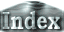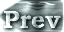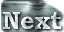### e. Runaway Greenhouse State

In the case of S=1800W/m2, integration over a long time period is not feasible in L32 even if Rayleigh friction and Newtonian cooling are included in the upper-most layer. This is probably attributable to two-grid noise arising in the vertical direction, amplifying significantly in magnitude and finally violating the CFL condition. Here, calculation results for the case with a single damping layer will be presented.

#### Time Evolution of Surface Temperature and OLR

 Figure 1 shows the time evolutions of the global mean values of surface temperature and OLR. In this figure, the results only up to Day 160 are presented because calculations for longer than 160 days are not feasible. However, OLR and surface temperature tend to decease and increase with time, respectively. Despite the fact that calculations can be made only for 160 days, the results from these 160 days suggest the initial emergence of a runaway greenhouse state. (a)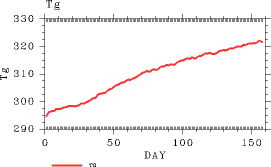(b)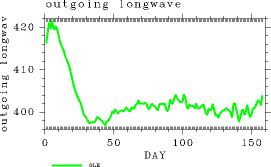Figure 1: Time evolutions of the global mean values. (a) Surface temperature (K) and (b) OLR (W/m2). The results are obtained from the atmospheric model with a single damping layer and S=1800 W/m2.

#### Two-Grid Noise

 As described above, it can be presumed that emergence of two-grid noise arising in the vertical direction is the direct reason that integration cannot be performed over a long time period by the model with a single damping layer. The amplitude of the noise increases in the upper atmospheric layer with time and eventually causes violation of the CFL condition, making time integration infeasible at the end of the model run. Figure 2 shows an example that captures the characteristics associated with such cases. The figure shows time evolutions of the vertical profiles of u and T at a point near the equator. Especially in the u-profile, patterns of two or three point cycle oscillations are evident. The values of temperature, T, drastically increase in the upper-most layer. It can be speculated that these characteristics are caused by gravity waves that propagate vertically from the troposphere, however, no analysis has yet been conducted to identify the cause of these characteristics. (a)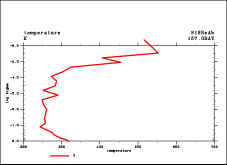(b)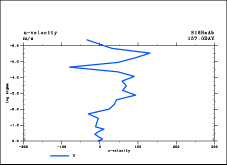Figure 2: Time evolution of the vertical structures for the case that includes Rayleigh friction and Newtonian cooling type smoothing only in the upper-most layer. (a) Temperature (K) and (b) the zonal wind (m/sec). The animation shows figures for a point at 2.7° latitude north for every 3 hours between Days 157 and 160. For solar constant, S=1800 W/m2 is used. The vertical axis shows all the heights included in the model domain in logσ. The horizontal axis in (a) shows temperature from 200 K to 700 K. The horizontal axis in (b) shows the zonal wind speed from -200 m/s to 300 m/s.

 D.e. Runaway Greenhouse State